Total: 71#### Perimeter, area, surface area and volume

This animation presents the formulas to calculate the perimeter and area of shapes as well as the surface area and volume of solids.#### Solids of revolution

Rotating a geometric shape around a line within its geometric plane as an axis results in a solid of revolution.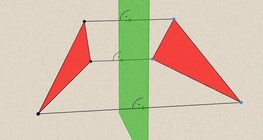#### Geometric transformations – reflection

This animation demonstrates geometric reflection, a type of geometric transformation both in plane and space.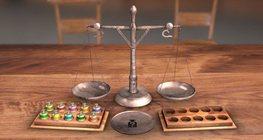#### Scale exercises

An interesting logical exercise: you have many weights that look the same, you have to find the only one that is different.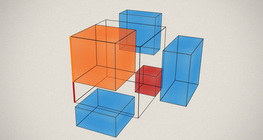#### Notable products

A spectacular demonstration of algebraic expressions.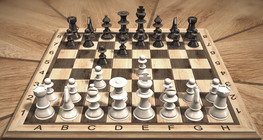#### Chess games

Chess games can help to develop logic skills.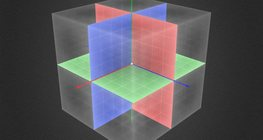#### Three-dimensional Cartesian coordinate system

3-dimensional Cartesian coordinate system with illustrations and exercises that develop spatial perception.#### Geometric transformations – rotation

This animation demonstrates geometric rotation, a type of geometric transformation both in plane and space.#### Geometric transformations – translation

This animation demonstrates geometric translation in both plane and space.#### Optical illusion

The information gathered by the eye is misinterpreted by the brain.#### Kepler´s laws of planetary motion

The three important laws describing planetary motion were formulated by Johannes Kepler.#### 3D puzzle

This exciting and colourful game is designed to develop spatial perception. You can check your solutions using isometric views.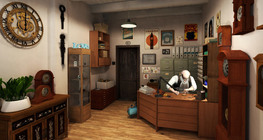#### What is the time?

A game to practise the use of analogue and digital clocks.#### Ames room

An Ames room is a distorted room that is used to create an optical illusion.#### Non-orientable surfaces

The Möbius strip and the Klein bottle are special two-dimensional surfaces with only one side.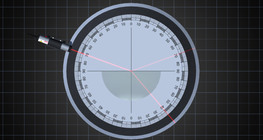#### The reflection and refraction of light

A ray of light is reflected or refracted at the boundary of two mediums with different refractive indices.#### Dividing Space into Regions by 3 Planes

Division of space by three intersecting planes in several different arrangements.#### Volume of a tetrahedron

To calculate the volume of a tetrahedron we start by calculating the volume of a prism.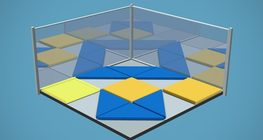#### 3D Tangram

A special, 3D version of the well-known Chinese logical game.#### Dice

Regular dice can be used for solving statistical and probability exercises.